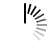# Scala Programming Examples

## Hello World

```object HelloWorld {
def main(args: Array[String]) {
println("Hello, world!")
}
}

```

## Compile and Execute Scala Online

```object HelloWorld {
def main(args: Array[String]) {
println(divide(-1, 2)(3, 4))
}

def divide(p: (Int, Int))(q: (Int, Int)): Either[String, (Int, Int)] =
{
def divTrueInp (x: (Int, Int)): Boolean =
if (Math.abs(x._1) < Math.abs(x._2)) false else true

var out = (p._1 * q._2, p._2 * q._1 )
var in = 0

if (out._1 > out._2) in = out._1
else in = out._2

for (i <- 1 to in) {
if (out._1 % i == 0 && out._2 % i == 0) {
val x1 = out._1 / i
val y1 = out._2 / i
out = (x1, y1)
}
}

if (p._2 == 0 || q._2 == 0 ) Left("Zero divisor")
else if (divTrueInp(p) || divTrueInp(q)) Left("Invalid input")
else if (divTrueInp(out) || out._2 == 0) Left("Improper result")
else Right(out)
}
}```

## 2019-03-22

```object HelloWorld {
def out(liste: List[Int]): Unit = {
if (liste != Nil) {
print(liste.head + ", ")
out(liste.tail)
}
}
def main(args: Array[String]): Unit = {
out(List(1,2,3))
}
}```

## Compile and Execute Scala Online

```object HomePage {
def main(args: Array[String]) {
println("Hello, world!")
}
}```

## Compile and Execute Scala Online

```class agescala{
def age{
var age:Int = 20;
if(age > 18){
println ("Age is greate than 18")
}
}
}

object Demo {
def main(args: Array[String]) {
var agobj = new agescala()
agobj.age
}

} ```

## HandsOn Scala

```object PatternMatching1
{

def main(args: Array[String])
{
def matchText(x : Any) : Any = x match
{

case 1 => "  One  "
case "two" => 2
case y: Int => " You have entered an integer >2   "
case _ => "many"

println(matchText(3))
println(matchText(2))
println(matchText("two"))
println(matchText(" Oops" ))

}

}

}```

## Compile and Execute Scala Online

```object HelloWorld {
def main(args: Array[String]) {
println(zipWith(List(1,2,3,4,5,6),List(1,2,3,4,5,6),(x: Int, y: Int) => x+y))

def zipWith[A,B,C](xs: List[A], ys: List[B], f: (A, B) => C): List[C] = {
val list = xs.zip(ys)
for (el <- list) yield f(el._1,el._2)
}
}
}```

## Compile and Execute Scala Online

```object HelloWorld {
def main(args: Array[String]) {
//I have a List(1 ,2 ,3 ,4 ,5) and trying to get a sublist: List(3, 4) from it by the following way:
val list = List(1 ,2 ,3 ,4 ,5)
//0, 1, 2, 3, 4
//3, 4
var b = list.splitAt(3)._2
println(b)
println(sum(list))
def sum(xs: List[Int]): Int = xs match {
case Nil => 0
case _ :: tail if tail.length==0 => 0 // size 1
case _ :: tail if tail.length==2 => tail(1) //size 2
case _ :: tail if tail.length>2 => tail(0) + tail(1) + sum(tail.splitAt(3)._2)
}
}
}```

## test

```object HelloWorld {
def main(args: Array[String]) {
println("Hello, world!")
}
}```

## Skype Interview with Zubko Zinovii, Middle Scala

```object HelloWorld {
def main(args: Array[String]) {
println("Hello, world!")
}
}```

AdvertisementsWe use cookies to provide and improve our services. By using our site, you consent to our Cookies Policy.